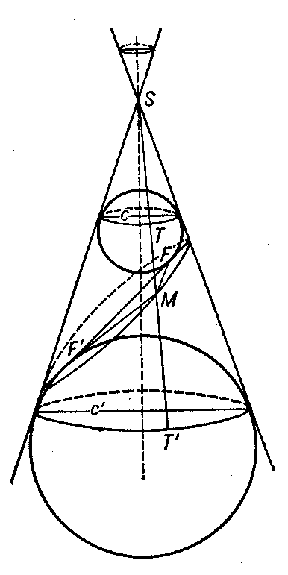# Dandelin spheres

Spheres forming part of the geometrical constructions relating the planimetric definition of the ellipse, hyperbola or parabola with their stereometric definitions. For instance, let two spheres (also known as Dandelin spheres), inscribed in a circular cone, make contact with the surface of the cone along circles $c$ and $c'$ (see Fig.) and let $\pi$ be a certain plane passing through two points $F$ and $F'$.If an arbitrary point $M$ is taken on the intersection line of the cone with $\pi$, and a generatrix $SM$ is drawn intersecting $c$ and $c'$, then if $M$ varies, the points $T$ and $T'$ move around the circles $c$ and $c'$ while preserving the distance $TT'$, i.e. the intersection line will be an ellipse ($MF'+MF=TT'$, $MF'=MT'$ and $MF=MT$). In the case of a hyperbola, Dandelin spheres are located in different sheets.